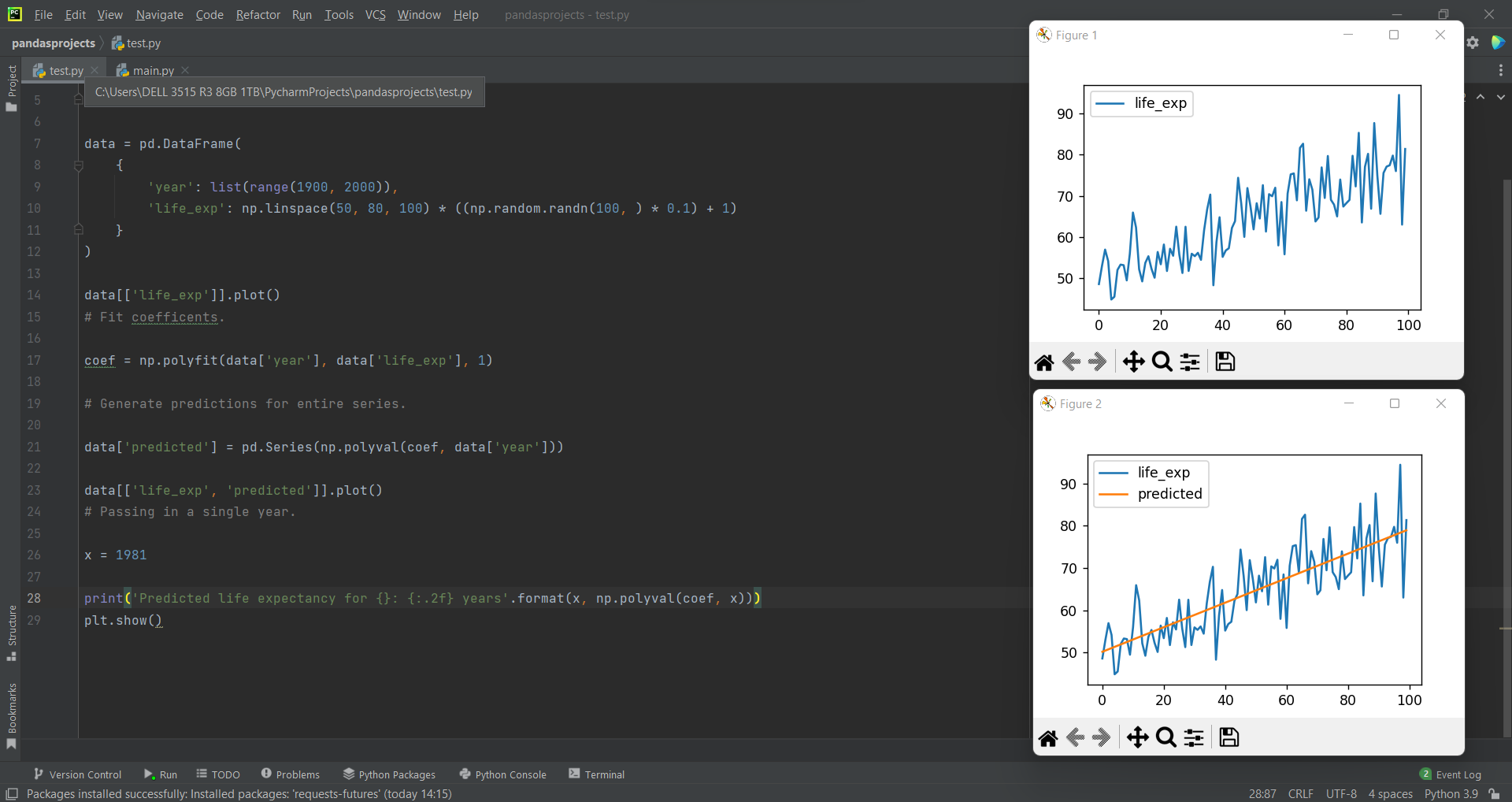# Predict a Value using Pandas Dataframeby Abdul Rawoof A RUpdated: Feb 20, 2023Solution Kit

In Python, predict() function enables us to predict the labels of the data values based on the trained mode, and the syntax for predict function is the model.predict(data). It accepts only a single argument, usually the data to be tested.

Predict function passes the input vector through the model and returns the output tensor for each data point, and since the last layer in your model is a single Dense neuron, then the output for any data point is a single value. We did not specify an activation for the last layer, and it will default to linear activation in Python. The predicted value is nothing but simulations that consider the estimation uncertainty and the fundamental uncertainty. For example, for any given of x, we go straight in the line and then move horizontally to the left of the data to discover the value of Y. The anticipated value of Y is called the predicted value of Y, and it is denoted Y'. Dependent variables are those we want to predict, whereas independent variables are variables we use to predict the other variable's value.

Libraries used to predict a value using Pandas DataFrame:

• Pandas: It is an open-source library that provides high-performance data manipulation in Python.
• Matplotlib: a comprehensive library for creating animated, interactive visuals and static.
• NumPy: It is a python library used for work with arrays.

Here is an example of how to predict a value using Pandas DataFrame:Fig : Preview of the output that you will get on running this code from your IDE.

### Code

In this solution we're using Pandas, Matplotlib and NumPy libraries.

### Instructions

Follow the steps carefully to get the output easily.

2. Copy the snippet using the 'copy' and paste it in your IDE.
3. Add required dependencies and import them in Python file.
4. Run the file to generate the output.

I hope you found this useful. I have added the link to dependent libraries, version information in the following sections.

I found this code snippet by searching for 'how to predict a value using pandas dataframe' in kandi. You can try any such use case!

### Environment Tested

I tested this solution in the following versions. Be mindful of changes when working with other versions.

1. The solution is created in PyCharm 2021.3.
2. The solution is tested on Python 3.9.7.
3. Pandas version-v1.5.2.
4. numpy version-v1.24.0.
5. Matplotlib version-v3.6.2.

Using this solution, we are able to predict a value using pandas dataframe with simple steps. This process also facilities an easy way to use, hassle-free method to create a hands-on working version of code which would help us to predict a value using pandas dataframe.

### Dependent Libraries

pandasby pandas-dev

Python37363Version:v2.0.0rc1Flexible and powerful data analysis / manipulation library for Python, providing labeled data structures similar to R data.frame objects, statistical functions, and much more

Support
Quality
Security
Reuse

pandasby pandas-dev

Python37363Version:v2.0.0rc1License: Permissive (BSD-3-Clause)

Flexible and powerful data analysis / manipulation library for Python, providing labeled data structures similar to R data.frame objects, statistical functions, and much more
Support
Quality
Security
Reuse

numpyby numpy

Python22989Version:v1.24.2The fundamental package for scientific computing with Python.

Support
Quality
Security
Reuse

numpyby numpy

Python22989Version:v1.24.2License: Permissive (BSD-3-Clause)

The fundamental package for scientific computing with Python.
Support
Quality
Security
Reuse

matplotlibby matplotlib

Python17007Version:v3.7.1License: No License (null)

matplotlib: plotting with Python

Support
Quality
Security
Reuse

matplotlibby matplotlib

Python17007Version:v3.7.1License: No License

matplotlib: plotting with Python
Support
Quality
Security